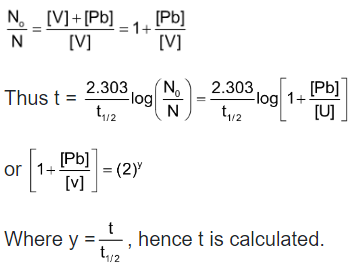## Chemical Kinetics of Class 12

The standard unit of radioactivity is curie (C) which is defined as that amount of any radioactive material which gives 3.7 × 1010 disintegrations per second (dps). i.e., 1C = 3.7 × 1010 dps.

Now a days, the unit curie is replaced by Rutherford (rd) which is defined as the amount of a radioactive substance which undergoes 106 dps; i.e., 1 rd = 106 dps.

1 C = 3.7 × 1010 dps = 37 × 103 rd

1 mC = 3.7 × 107 dps = 37 rd

However, in SI system the unit of radioactivity is becquerel (Bq)

1Bq = 1 disintegration per second

106 Bq = 1 rd

3.7 × 1010 Bq = 1 C

According to the theory of radioactive disintegration, the product of a radioactive disintegration may also be radioactive and the rate of disintegration of the daughter element depends upon the amount of it present at different time. When steady state is reached,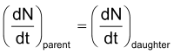or, λ1N1 = λ2N2

Where λ1 and λ2 are the disintegration constants for the decay of parent and daughter radioactive species and N1 and N2 are the number of atoms disintegrating at a given time.

Thus,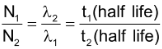Activity: Activity is the rate at which the radioactive substance decays

Activity = λ×N =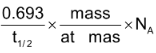Specific Activity: Activity of the unit mass of radio isotope.

Maximum yield of daughter element

Let A → B (daughter element)

If λA and λB are decay constants of ‘A’ and ‘B’. Maximum activity time of daughter element can be calculated as:

tmax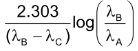Number of atoms of daughter element B after time t:

[NB] =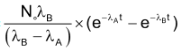Nuclear Fission: It is a nuclear reaction in which a heavy nucleus splits into lighter nuclei and energy in released.

⇒ Californium - 252 decays both by alpha emission (97%) and by spontaneous fission (3%). During spontaneous fission, the nucleus splits into two stabler nuclei plus several neutrons.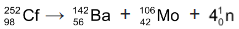⇒ When a neutron strikes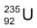nucleus, the nucleus splits into roughly equal parts, giving off several neutrons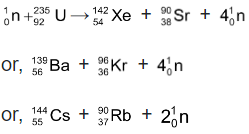⇒ If the neutrons from each nuclear fission are absorbed by other uranium - 235 nuclei, these nuclei split and release even more neutrons. Thus a chain reaction can occur.

Spallation: Bombardment with high energy charged particles can break some target nuclei into several smaller nuclei with the emission of a large number of nucleus (10 to 20 or more). These are called spallation reaction.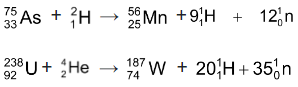Nuclear fusion: In this type of nuclear reaction, certain light nuclei may fuse together with the liberation of tremendous amount of energy. To achieve this, the colliding nuclei must possess enough kinetic energy to overcome the initial repulsion between the positively charged cores. This energy may be made available by raising the temperature of the reacting system to several million degress. Such reactions are therefore also known as thermonuclear reactions.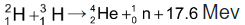The energy of a fusion process is due to mass defect (converted to Binding Energy). The high temperature required to initiate such reactions may be attainted initially through a fission process.

Artificial Radioactivity: Bombardment of stable elements with high energy α-particles, protons, neutrons, deutrons or γ-rays produce radioactive nuclide. These radionuclide do not occur naturally and may be called man - made or artificial. The radioactivity exhibited by these artificial radio nuclide is referred to as artificial radioactivity or as induced radioactivity.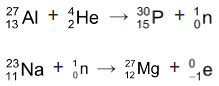### Binding Energy and Packing fraction

The nearest integer to the mass of a nuclide is called the mass number of that isotope. The difference between the actual isotopic mass and mass number is termal as mass defect of the nuclide.

Thus Δm (mass defect) = isotopic mass - mass number

Aston introduced a term "Packing fraction" for each nuclide to compare their mass excess, it was defined as:

Packing fraction (f)=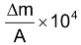Binding energy (B) = Δm × c2

Binding energy of the nucleus is equal to the energy required to split the nucleus into its component nucleons. Nuclear binding energy is generally expressed in MeV (mega - electron volt).

Since 1 a.m.u. = 1.66 × 10–27 Kg

∴ (B) for one a.m.u. = 1.66 × 10–27 × (3×108)2J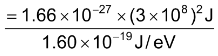= 931 × 106 eV = 931 MeV

∴ (B) = ΔM (in a.m.u.) × 931 MeV

Binding energy per nucleon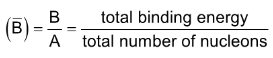(i) Radio Carbon Dating: The application of the C - 14 dating is based on the fundamental assumption that the intensity of cosmic ray and hence of 14C in the atmosphere has been remaining constant over many thousands of years. This gives the initial activity of C - 14 corresponding to the time when the plant or animal died and assimilation of radio active carbon ceased to continue.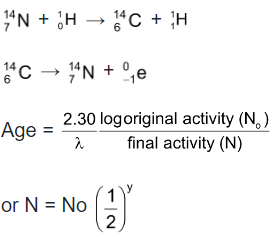where y = t/t1/2

(ii) Rock Dating: It is based on the kinetics of radioactive decay. It is assumed that no lead was initially in the sample and the whole of it came from the uranium.

Initial radioactivity = [U] = N0 in terms of gm atoms

Final radioactivity = [U] = N

We have assumed that due to high value of tx/2 of uranium its amount remains unchanged.